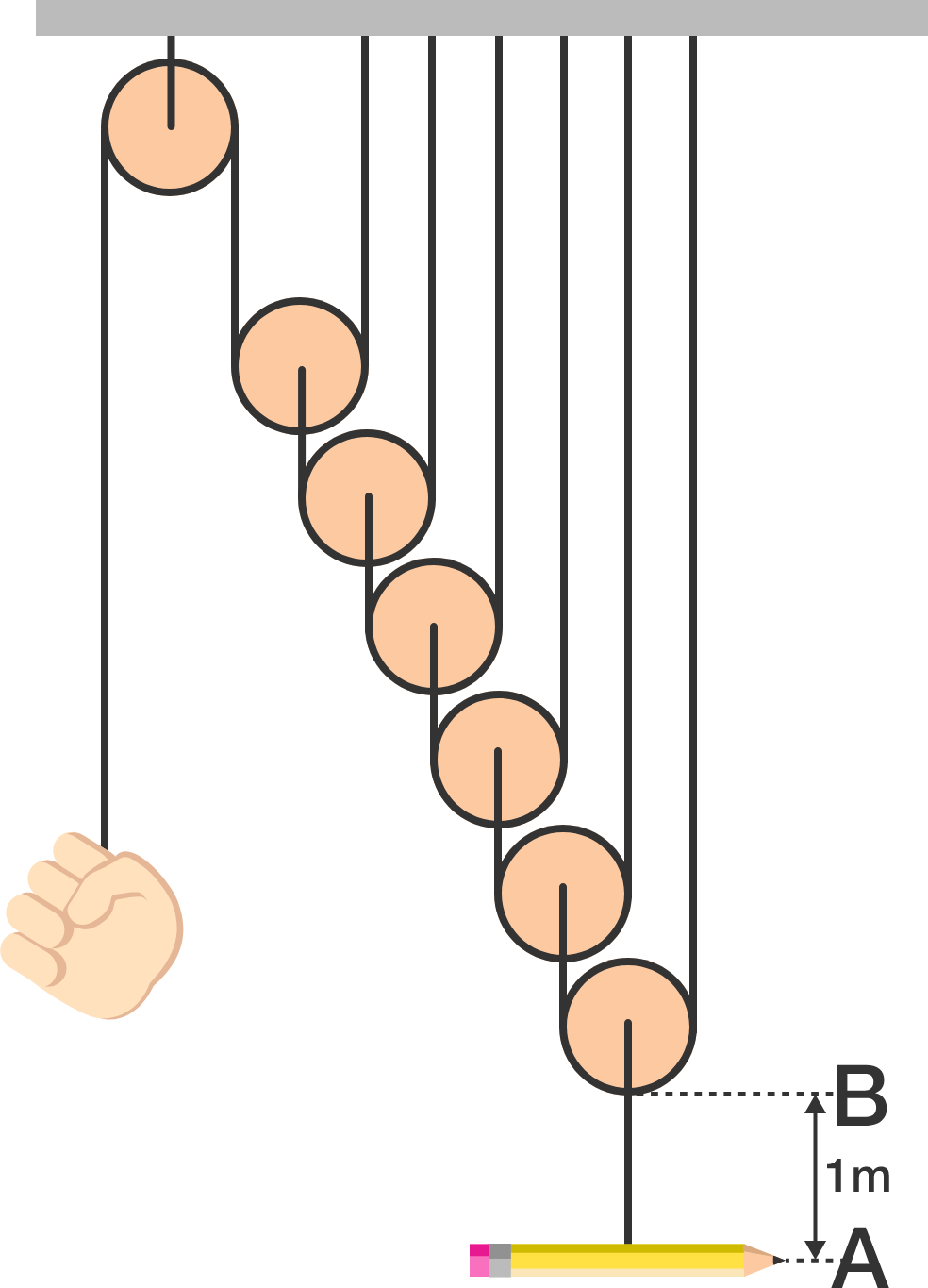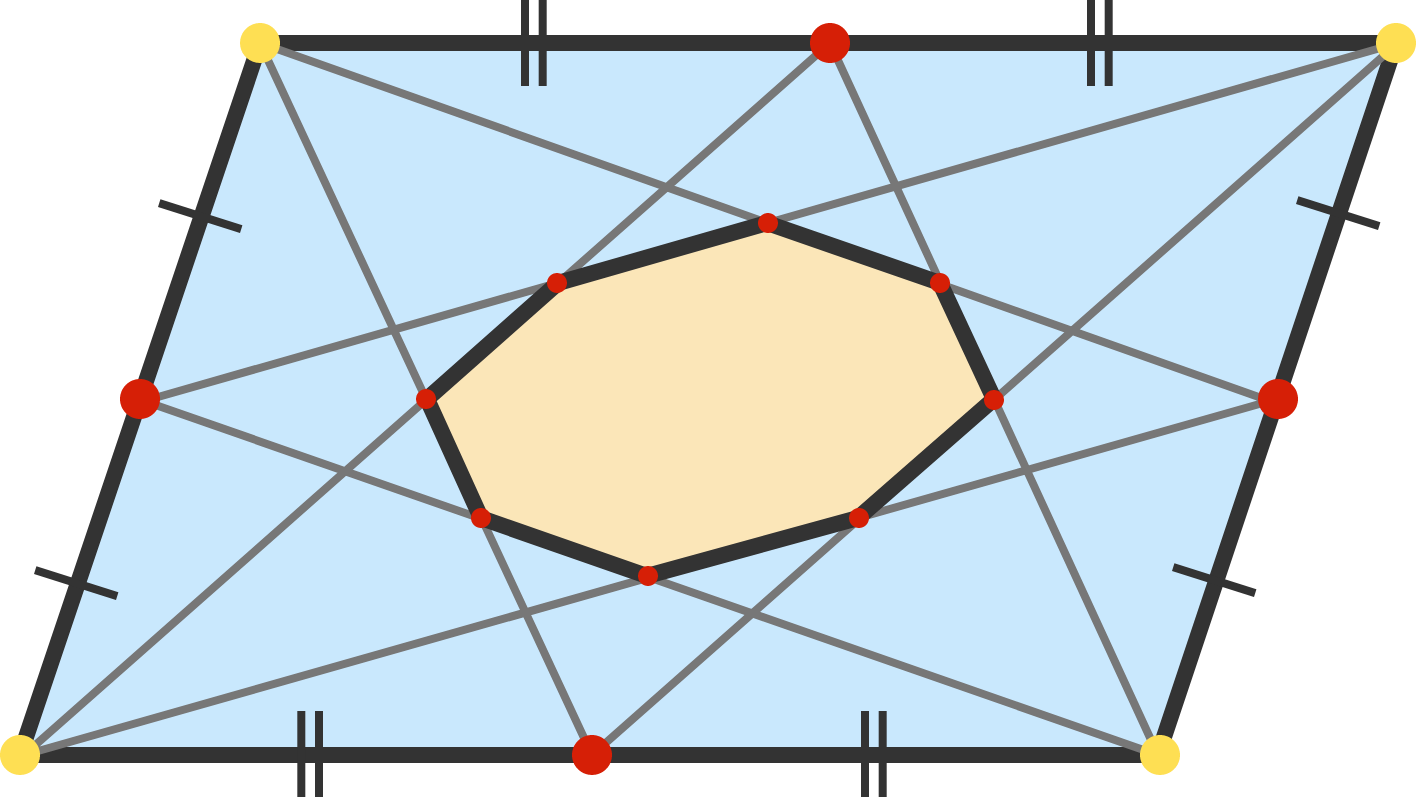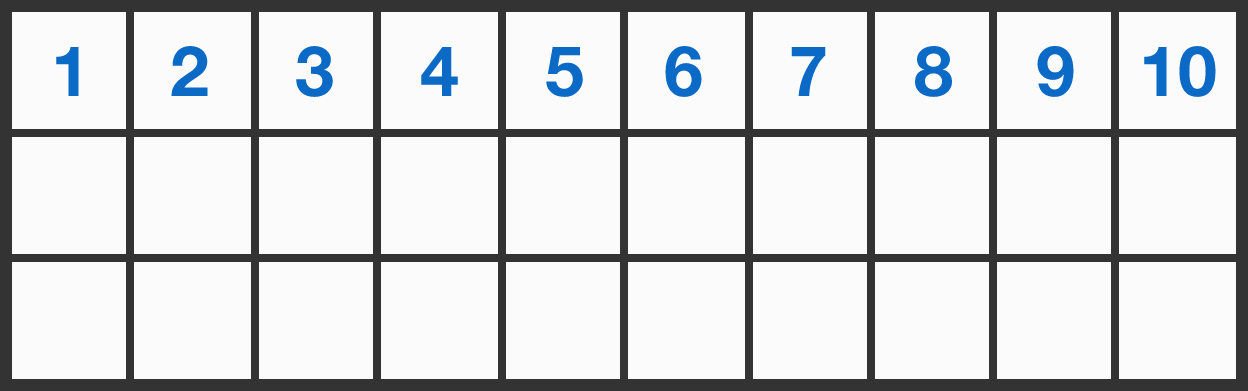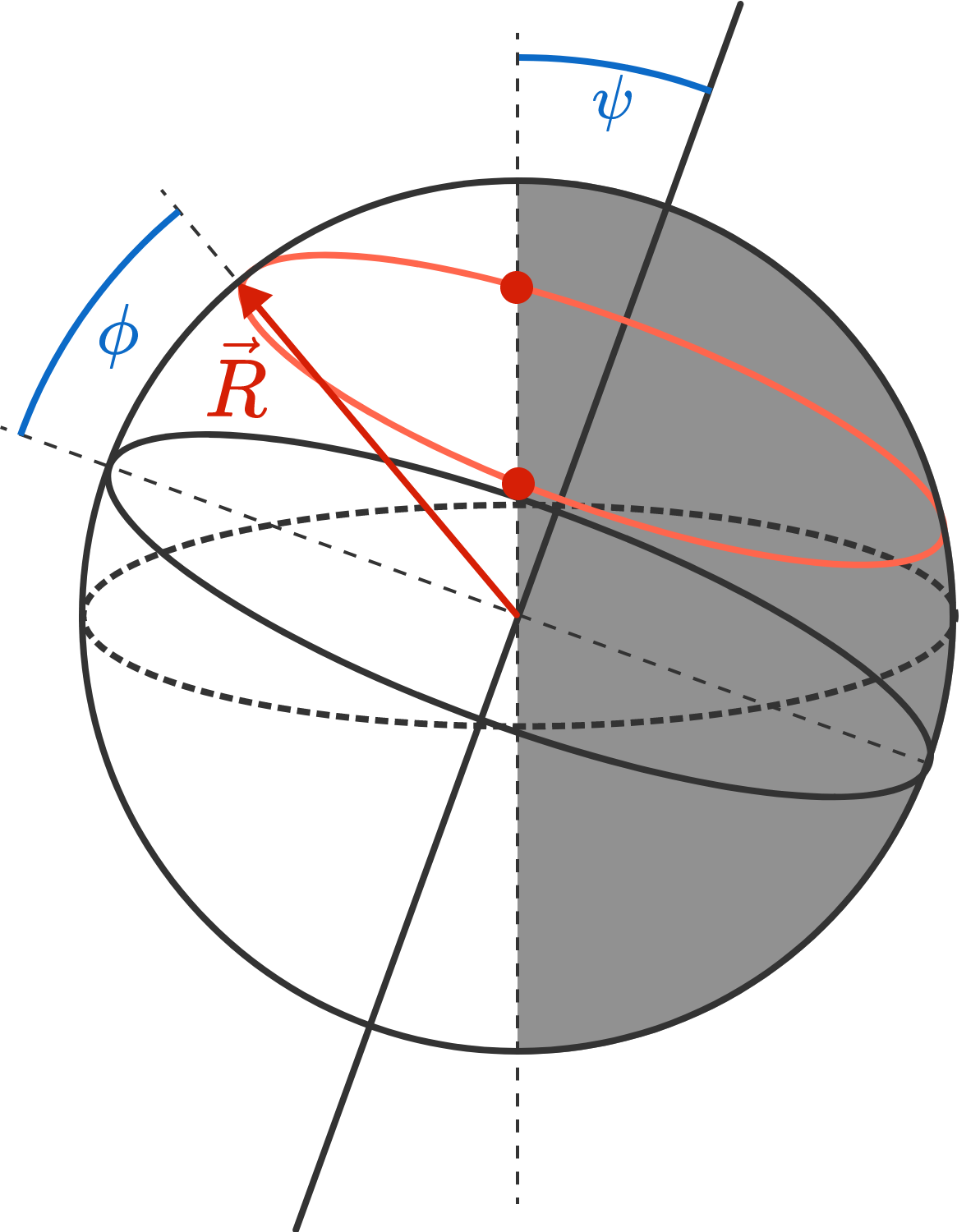# Problems of the Week

Contribute a problem

# 2017-11-06 IntermediateNote: the rope lengths are NOT drawn to scale.

How far (in meters) should the hand pull the rope to move the pencil from A to B?

Assume that the hand pulls the rope straight down and that the pulleys are far enough apart that they do not collide with each other.Given any parallelogram, draw two line segments from each corner to connect to the midpoints of the opposite sides. These eight line segments form an octagon, as shown above.

Let $O$ be the octagon's area and let $P$ be the parallelogram's area. To three decimal places, what is $\frac{O}{P}?$The $3\times 10$ grid above is to be filled with integers in the following way:

• The second row is completed with the numbers from 1 to 10 in any order.
• Each number in the third row is the sum of the two numbers above it.

Is there any way to complete the second row so that the units digits of the numbers in the third row are all different?

Alyssa, Bill, and Clayton are playing ping pong. The rules are as follows:

1. The first game is between Alyssa and Bill.

2. Whenever a game ends, the next game is played with the winner against the person who was sitting out.

3. No ties are possible.

For example, one possible sequence of results is as follows:

Alyssa defeats Bill.

Clayton defeats Alyssa.

Clayton defeats Bill.

Alyssa defeats Clayton.

After some number of games have been played, they count how many times each player won; Alyssa won 10 times and Bill won 21 times.

How many times did Alyssa and Bill play against each other?Approximately how long is the daytime (the time between sunrise and sunset) on the $21^\text{st}$ of December in Paris?

Note: Paris is on the latitude $\phi = 49^\circ.$ The Earth is tilted at an angle of $\psi = 23.4^\circ$ relative to its axis of rotation.

×